# Beginning Algebra Review Exam 1## Material covered: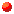Real Arithmetic and Subsets of the Real Numbers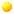Order of Operations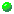Translating Phrases into Expressions and Sentences into Equations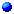Solving Equations and Problem Solving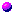Combining Like Terms and Simplifying Expressions

```1.  List the word or words found in your text to indicate the following operations:
```2.  Arrange the following four elements to match the order of operations given
a.  Addition and subtraction (left to right)
b.  Multiplication and division (left to right)
c.  Exponentiation
d.  Any operation inside a symbol of grouping or above or below a fraction bar```
```3.  Be able to translate phrases into algebraic expressions.  Examples:
a.  The sum of two and x divided by three times y
b.  Five divided by x decreased by two
c.  Twice x divided by the sum of three and z
d.  Three times the sum of x and 2```
```4.  Be able to translate sentences into equations.  Examples
a.  If twice a number is increased by 2 the sum is
fourteen.
b.  If the quotient of y divided by 3 is increased by
2 the sum is 7.
c.  The sum of twice an integer and the integer itself
is equal to the integer plus 14.
d.  If three is subtracted from the sum of y and z
the result is 12.```
```5.  Be able to set up an equation in a single variable to solve each of the following.
Be sure to state what the variable represents in each problem.
a.  Tom's age is three times Mary's age.  If the sum of
their ages is 52, what is Mary's age.
b.  Jane buys a computer which costs \$1350 and agrees to pay for
the computer on time.  She pays \$150 down and agrees
to pay the balance in 12 equal monthly payments.  Find the
amount of each of the monthly payments.
c.  A cold front hit and the temperature fell to 13 degrees, a drop of
15 degrees in thirty minutes.  What was the temperature before
front hit?
d.  One number is six more than twice the other, and their sum is 69.
Find the numbers.```
```6.  Evaluate:

a.  -16/8 - 4

b.  [12 - (-3)(-2)]/[-7 - 3(-1) + 1]

c.  6 - (2 + [ 5 - (-4) ] + (-7) )

d.  12/6 - 2(5)

e.  3/4 + 2/6

f.  -2/5 - 1/3

g.  [-7/15][3/4]

h.  Divide 4/9 by 5/3.```
```7.  Solve for x:

a.  x - 7 = -5		b.  -3x + 7 = 13

c.  4x + 1 = -5		d.  x/2 - 3 = -8

e.  2(x - 1) + 3x = 8```
```8.  Simplify.

a.  2x² - 3y + 7x² + 10y

b.  (3x - 5) - (-2x + 7)

c.  5(3x - 2) + 4(x + 3)```
```Give an example of

a.  A real number that is not rational.

b.  A rational number that is not an integer.

c.  An integer that is not a whole number.

d.  A whole number that is not a natural number.
```